# Search

About 14 Search Results Matching Types of Worksheet, Worksheet Section, Generator, Generator Section, Kids Sudoku Puzzle3, Subjects matching Math, Type matching Worksheet Section, Grades matching 4th Grade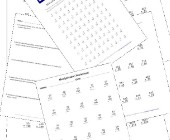## Multiplication Worksheets for Kids

This set of math worksheets is geared towards h...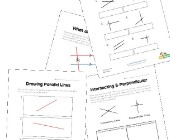## Parallel, Perpendicular and Intersecting Lines Worksheets

Help kids learn about lines including parallel ...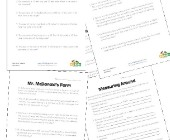## Area and Perimeter Word Problems

This collection of area and perimeter worksheet...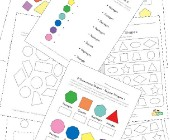## Geometry Worksheets

Help kids learn the early basics of geometry in...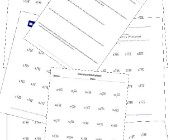## Division Worksheets

Help kids learn division with this collection o...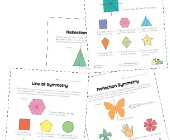## Symmetry Worksheets

Help kids learn about the concept of symmetry w...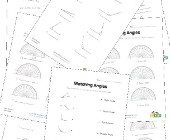## Angles Worksheets

Help kids learn about angles with this collecti...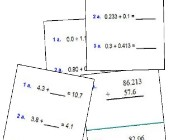Help kids practice decimal addition with the fo...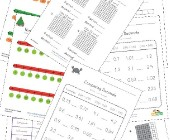## Decimal Worksheets

This collection of free decimal worksheets will...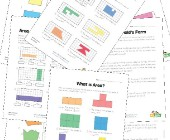## Area and Perimeter Worksheets

Help kids learn to calculate the area and perim...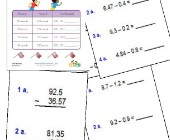## Subtracting Decimals Worksheets

These worksheets will help children practice de...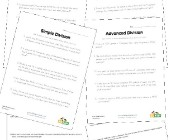## Division Word Problems

Practice division and problem solving with thes...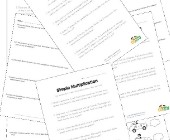## Multiplication Word Problems

Practice multiplication and problem solving wit...